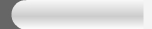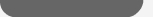Copyright (c) Newday Com. Ltd. All rights reserved-2001-2008### By the end of this lesson, learners should be able to:

• Understand the parts of an Excel formula
• Create a simple addition formula
• Create a simple subtraction formula using the Point-and-Click Method
• Create simple multiplication formulas
• Create simple division formulas

### About Formulas

In school, you learned formulas used to calculate math problems. Microsoft Excel uses these same formulas to perform calculations in a spreadsheet.

A formula can be a combination of values (numbers or cell references) and math operators (+, -, /, *, =) into an algebraic expression. Excel requires every formula to begin with an equal sign (=).

The following table illustrates the mathematical operators learned in school and those represented in Excel 2003.

 School Excel 2003 Addition + + Subtraction - - Multiplication X * Division / / Equals = =

The result of a formula-the answer to 2+3, for example-displays in the cell on the Excel worksheet. The formula is visible only in the formula bar. A formula's result will change as different numbers are entered into the cells included in the formula's definition.### Creating a Simple Addition Formula

A simple formula in Excel contains one mathematical operation only: one number plus a second number equals a third number. Writing a simple formula is really no more difficult than that: 1+1. The only difference in Excel is that all formulas must begin with the equal sign (=). It is not enough to type 1+1 in Excel because what will appear in the cell is "1+1." You must begin the equation with an equal sign, or =1+1. This holds true for any formula, simple or complicated, that adds, subtracts, multiplies or divides.

Let's add two numbers to create a third, 128+345=473. In Excel, this would be expressed by the formula, =128+345, as shown below.##### To Create a Simple Formula that Adds Two Numbers:
• Click the cell where the formula will be defined.
• Type the equal sign (=) to let Excel know a formula is being defined.
• Type the first number to be added (128, for example)
• Type the addition sign (+) to let Excel know that an add operation is to be performed.
• Type the second number to be added (345, for example
• Press Enter or click the Enter button on the Formula bar to complete the formula.

### Creating a Simple Addition Formula (continued)

But what if a column contains many numbers, each of which regularly changes? You don't want to write a new formula each time a number is changed. Luckily, Excel 2003 lets you include cell references in formulas.

A formula can add the value of two cells-B2 and B3, for example. Type any two values in these two cells and the formula will adjust the answer accordingly.

Using this method to calculate two numbers-128 and 345, for example-requires that you type 128 in cell B2, for example, and 345 in cell B3. The Excel formula, =B2+B3, would then be defined in cell B4.##### To Create a Simple Formula that Adds the Contents of Two Cells:
• Type the numbers you want to calculate in separate cells (for example, type 128 in cell B2 and 345 in cell B3).
• Click the cell where the answer will appear (B4, for example).
• Type the equal sign (=) to let Excel know a formula is being defined.
• Type the cell number that contains the first number to be added (B2, for example).
• Type the addition sign (+) to let Excel know that an add operation is to be performed.
• Type the cell number that contains the first number to be added (B3, for example).
• Press Enter or click the Enter button on the Formula bar to complete the formula.

### Creating a Simple Subtraction Formula Using the Point-and-Click Method

Formulas can be created by using either numbers or cell references in the definition. You can also use the mouse to select the cells to be used in the formula instead of typing the cell number or cell reference. Using this method, we are going to write a simple formula that subtracts one cell from another: =B3-B2.##### To Create a Simple Formula using the Point and Click Method:
• Type the numbers you want to calculate in separate cells (for example, type 128 in cell B2 and 345 in cell B3).
• Click the cell where the answer will appear (B4, for example).
• Type the equal sign (=) to let Excel know a formula is being defined.
• Click on the first cell to be included in the formula (B3, for example).
• Type the subtraction sign (-) to let Excel know that a subtraction operation is to be performed.
• Click on the next cell in the formula (B2, for example).
• If you include multiple cells in the formula, repeat steps 4 and 5 until the entire formula is entered.
• Press Enter or click the Enter button on the Formula bar to complete the formula.

### Creating Simple Multiplication Formulas

Creating multiplication formulas is very similar to addition and subtraction formulas. To multiply two cells the formula, B2 and B3, you would need to insert a multiplication operator * between them, =B2*B3.##### To Create a Simple Formula that Multiplies the Contents of Two Cells:
• Type the numbers you want to calculate in separate cells (for example, type 128 in cell B2 and 345 in cell B3).
• Click the cell where the answer will appear (B4, for example).
• Type =
• Click on the first cell to be included in the formula (B2, for example).
• Type a mathematical operator (Ex: the multiplication symbol *). The operator displays in the cell and Formula bar.
• Click on the next cell in the formula (B3, for example).
• If you include multiple cells in the formula, repeat steps 4 and 5 until the entire formula is entered.
• Press Enter or click the Enter button on the Formula bar to complete the formula.

### Creating Simple Division Formulas

Creating division formulas is very similar to the addition, subtraction and multiplication formulas. To divide the contents of cell B2 by cell B3, you would need to insert a division operator / between them, =B2/B3.

##### To Create a Simple Formula that Divides One Cell by Another:• Type the numbers you want to calculate in separate cells (for example, type 128 in cell B2 and 345 in cell B3).
• Click the cell where the answer will appear (B4, for example).
• Type the equal sign (=) to let Excel know a formula is being defined.
• Click on the first cell to be included in the formula (B2, for example).
• Type a mathematical operator (Ex: the division symbol /). The operator displays in the cell and Formula bar.
• Click on the next cell in the formula (B3, for example).
• If you include multiple cells in the formula, repeat steps 4 and 5 until the entire formula is entered.
• Very Important: Press Enter or click the Enter button on the Formula bar. This step ends the formula.

### Challenge!

• Open your Monthly Budget file.
• Add cells C2 through C10 using a handheld calculator, the calculator on your computer, or pencil and paper.
• If you included additional monthly bills in cells C11 through 14, add cells C2 through C14 together to get your total monthly expenses.How long did it take you to add all those numbers? Well, in the next three modules you will learn how Excel can do the math for you!

• Type the total you came up with in cell C15.
• Type a subtraction formula in C17 that subtracts the amount in C15 from the amount in C16.
• Save and close the Monthly Budget file.

 NOTICE BOARD 1  Student should make sure to login to class discussion board to view the neccessary information about his or her progress.  Don,t forget to log out when you are about to leave the classNOTICE BOARD 2 Students Should not forget to send his or her registration password and User name to newday.com@gmail.com For future refrence.NOTICE BOARD 3 Student should make sure to subscribe to newsletter of this site for useful information.MORE ADVANCE COURSE  Microsoft Access  Peachtree Account  Adobe Photoshop  ProgrammingENGINNERING COURSE  Café Management  Repair and Maintenance  Electronic Basic  Networking